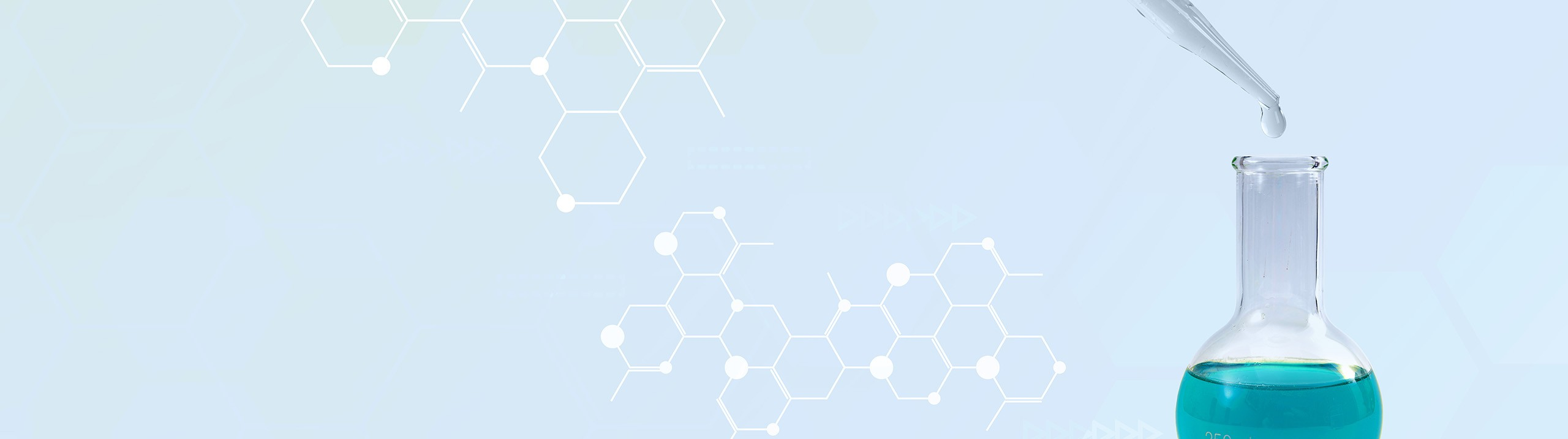## Analytical Chemistry

Learn the toughest concepts covered in your Analytical Chemistry class with step-by-step video tutorials and practice problems.

8. Monoprotic Acid-Base Equilibria

# Bronsted-Lowry Acids and Bases

Bronsted-Lowry Acids and Bases
1
concept

## Bronsted-Lowry Acid-Base3m
Play a video:
2
example

## Bronsted Acid-Base1m
Play a video:
So here we have to write the formula of the conjugate base for the following compound. So here conjugate base means that this is acting as an acid. So if we want to write its conjugate base, that means we have to remove NH plus from the compound itself. Remember if they're asking for the conjugate base? Just removing H Plus from the compound and you'll have your conjugate base. So here removing an H. Plus from it gives us S. +03. Remember we're not only losing a hydrogen but we're losing a positive charge here, it's already negative one. Losing a positive charge means it becomes more negative. So becomes S. +032 minus. In actuality what's going on here is we have a chess. So three minus reacting with water, it is acting as the acid, water is acting as the base. So it's going to donate an H plus away and that's how it becomes S. +032 minus. In the process, water accepts an H. Plus to become a church +30. Plus here, sulfate ion would represent the conjugate base of hydrogen sulfate sulfate or by sulfate which is H. S. +03 minus now that you've seen that example. I think of it the opposite way in which we have to find the contra acid of the example given below. Um Once you do that, click on the next video and see if your answer matches up with mine
3
example

## Bronsted Acid-Base1m
Play a video:
So here we have to write the conjugate acid for the compound given. So if we want its conjugate acid, that must mean that this is it acts as a base and accepts an H. Plus. So here by accepting an H. Plus it becomes H two P. 03. Here, you're not only accepting a hydrogen, but you're accepting a plus one charge as well, its charges already two minus. By accepting a plus one, it becomes more positive. So now it's H two P. +03. What's happening is that it's reacting with water? It is acting as the base now, so water is acting as the acid. Water is gonna donate an H. Plus to it. That's how it becomes a church to P. +03 minus. And in the process by losing an H. Plus, water becomes O. H minus. So we'd say here that this is called di hydrogen, phosphate. H two P 03 minus would represent the conjugate acid of our starting material of HP oh 32 minus. Now that we've seen those two examples, put it all together and classify each one of the following compounds under the words provided. Once you do that, come back and see how your answer matches up with mine
4
example

## Bronsted Acid-Base1m
Play a video: